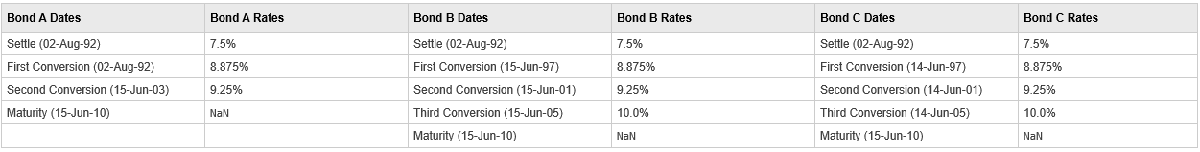# stepcpnyield

Yield to maturity of bond with stepped coupons

## Syntax

``Yield = stepcpnyield(Price,Settle,Maturity,ConvDates,CouponRates)``
``Yield = stepcpnyield(___,Period,Basis,EndMonthRule,Face)``

## Description

example

````Yield = stepcpnyield(Price,Settle,Maturity,ConvDates,CouponRates)` computes the yield to maturity of bonds with stepped coupons given the price. The function supports any number of conversion dates.```

example

````Yield = stepcpnyield(___,Period,Basis,EndMonthRule,Face)` adds additional optional arguments.```

## Examples

collapse all

Find the yield to maturity of three stepped-coupon bonds of known price, given three conversion scenarios:

• Bond A has two conversions, the first one falling on the settle date and immediately expiring.

• Bond B has three conversions, with conversion dates exactly on the coupon dates.

• Bond C has three conversions, with one or more conversion dates not on coupon dates. This case illustrates that only cash flows for full periods after conversion dates are affected, as illustrated below.The following table illustrates the interest-rate characteristics of this bond portfolio.Define the specifications for the bonds.

```format long Price = [117.3824; 113.4339; 113.4339]; Settle = datenum('02-Aug-1992'); ConvDates = [datenum('02-Aug-1992'), datenum('15-Jun-2003'), nan; datenum('15-Jun-1997'), datenum('15-Jun-2001'), datenum('15-Jun-2005'); datenum('14-Jun-1997'), datenum('14-Jun-2001'), datenum('14-Jun-2005')]; Maturity = datenum('15-Jun-2010'); CouponRates = [0.075 0.08875 0.0925 nan; 0.075 0.08875 0.0925 0.1; 0.075 0.08875 0.0925 0.1]; Basis = 1; Period = 2; EndMonthRule = 1; Face = 100;```

Use `stepcpnyield` to compute the yield to maturity for the bonds with stepped coupons.

`Yield = stepcpnyield(Price, Settle, Maturity, ConvDates, CouponRates, Period, Basis, EndMonthRule, Face)`
```Yield = 3×1 0.072214402049150 0.072214267800360 0.072864799557221 ```

## Input Arguments

collapse all

Price of bond, specified as a scalar or `NUMBONDS`-by-`1` vector of numeric values.

Data Types: `double`

Settlement date, specified either as a scalar or `NUMBONDS`-by-`1` vector of serial date numbers.

`Settle` must be earlier than `Maturity`.

Data Types: `double`

Maturity date, specified as a scalar or an `NUMBONDS`-by-`1` vector of serial date numbers representing the maturity date for each bond.

Data Types: `double`

Conversion dates, specified as a `NSTP`-by-max(`NCONV`) matrix containing conversion dates after `Settle`. The size of the matrix is equal to the number of instruments by the maximum number of conversions. Fill unspecified entries with `NaN`.

Data Types: `double`

Bond coupon rate, specified as an `NSTP`-by-max(`NCONV+1`) matrix containing coupon rates for each bond in the portfolio in decimal form. The matrix size is equal to the number of instruments by maximum number of conversions + 1. First column of this matrix contains rates applicable between `Settle` and the first conversion date (date in the first column of `ConvDates`). Fill unspecified entries with `NaN`

`ConvDates` has the same number of rows as `CouponRates` to reflect the same number of bonds. However, `ConvDates` has one less column than `CouponRates`. This situation is illustrated by

```Settle---------ConvDate1-----------ConvDate2------------Maturity Rate1               Rate2                 Rate3```

Data Types: `double`

(Optional) Coupons per year, specified as an `NUMBONDS`-by-`1` vector. Values for `Period` are `1`, `2`, `3`, `4`, `6`, and `12`.

Data Types: `double`

(Optional) Day-count basis of each instrument, specified as an `NUMBONDS`-by-`1` vector.

• 0 = actual/actual

• 1 = 30/360 (SIA)

• 2 = actual/360

• 3 = actual/365

• 4 = 30/360 (PSA)

• 5 = 30/360 (ISDA)

• 6 = 30/360 (European)

• 7 = actual/365 (Japanese)

• 8 = actual/actual (ICMA)

• 9 = actual/360 (ICMA)

• 10 = actual/365 (ICMA)

• 11 = 30/360E (ICMA)

• 12 = actual/365 (ISDA)

• 13 = BUS/252

Data Types: `double`

(Optional) End-of-month rule flag for generating dates when `Maturity` is an end-of-month date for a month having 30 or fewer days, specified for each bond as a nonnegative integer [`0`, `1`] using a `NUMBONDS`-by-`1` vector.

• `0` = Ignore rule, meaning that a payment date is always the same numerical day of the month.

• `1` = Set rule on, meaning that a payment date is always the last actual day of the month.

Data Types: `logical`

(Optional) Face value, specified for each bond as an `NUMBONDS`-by-`1` vector of nonnegative face values.

Data Types: `double`

## Output Arguments

collapse all

Yield to maturity, returned as a `NUMBONDS`-by-`1` vector in decimal form.

Note

For bonds with fixed coupons, use `bndyield`. You receive the error ```incorrect number of inputs``` if you use a fixed-coupon bond with `stepcpnyield`.